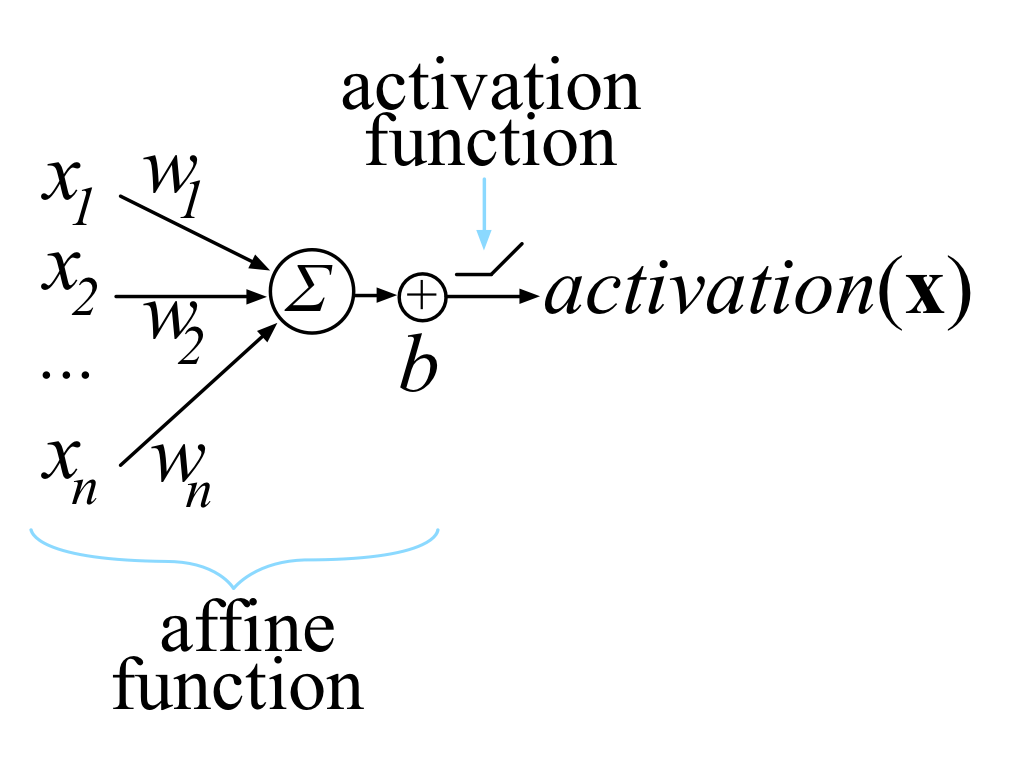# The matrix calculus you need for deep learning

0

## The matrix calculus you need for deep learning

### The matrix calculus you need for deep learningMost of us last saw calculus in school, but derivatives are a critical part of machine learning, particularly deep neural networks, which are trained by optimizing a loss function. This article is an attempt to explain all the matrix calculus you need in order to understand the training of deep neural networks. We assume no math knowledge beyond what you learned in calculus 1, and provide links to help you refresh the necessary math where needed.

February 23, 2020
Subscribe
Notify of